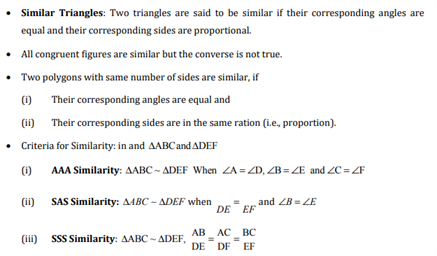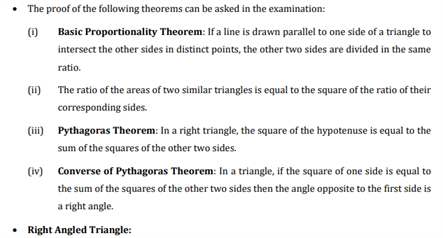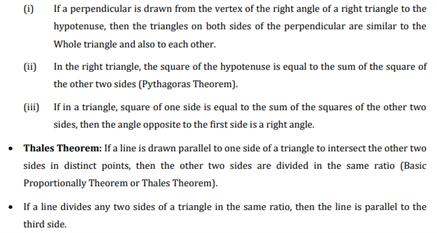# Revision Notes For CBSE Class 10 Maths Chapter 6 Triangles

Students might already be familiar with the topic of triangles and many of their properties in earlier classes. However, in class 10, the topic is discussed in more detail and students are further introduced to more complex concepts like similar figures, similarity of triangles, the relation between Pythagoras theorem and more. To facilitate better learning and help students explore these concepts in an easy way, CBSE revision notes for class 10 maths chapter 6 – triangles are available here. Students can download the free PDF to develop a clear understanding of the whole chapter and use it as an effective tool to complete assignments as well as study for class tests or exams.

Have a look at class 10 maths chapter 6 CBSE revision notes PDF below.Access CBSE Class 10 Maths Sample Papers Here.

Access NCERT Class 10 Maths Book Here.